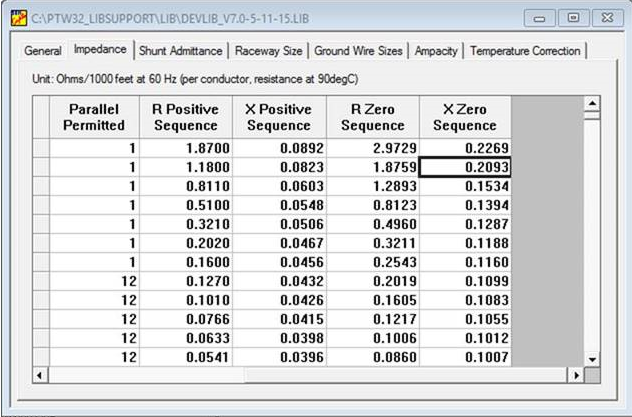• Sales
• Library Model Request
• Technical Support

# Zero Sequence Impedance in Cables

When the R and X Positive Sequence Impedances are entered in a cable library, the R and X Zero Sequence cells also get populated.What equations/formula are used to calculate these values?

Factor for RZERO_NONMAG = 1.5898;
Factor for XZERO_NONMAG = 2.5442;
Factor for RZERO_MAG = 3.1515;
Factor for XZERO_MAG = 2.4617;

If DuctMaterial is "Non-Magnetic"
Rzero = 1.5898 *Rpos;
Xzero = 2.5442 *Xpos;

If DuctMaterial is "Magnetic"
Rzero = 3.1515 *Rpos;
Xzero = 2.4617 *Xpos;
Creation date: 2/13/2017 10:44 AM      Updated: 4/30/2018 1:44 PM